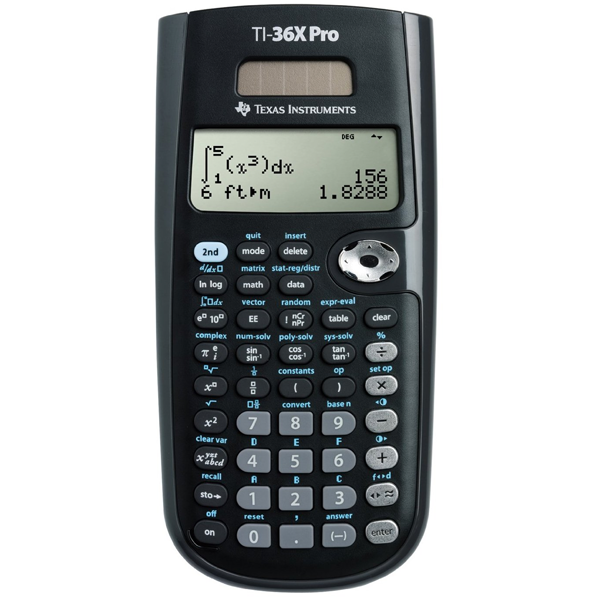# TI 36X Pro Scientific CalculatorSKU:
1070300

# TI 36X Pro Scientific Calculator

 \$20.95
Description:

### Key features:

• Four-line display
• One- and two-variable statistics
• MultiView display shows multiple calculations at the same time on screen
• Select degrees/radians, floating/fix, number format modes
• Choose from three solvers: numeric equation, polynomial and system of linear equations
• Display a defined function in a tabular form
• Determine the numeric derivative and integral for real functions
• Perform vectors and matrices using a vector and matrix entry window
• Exam acceptance: The TI-36X Pro scientific calculator is approved for use on SAT, ACT, and AP exams.

### Ideal for:

• Algebra 1 and 2
• Geometry
• Trigonometry
• Statistics
• Calculus
• Biology
• Chemistry
• Physics
• Computer Science
• College math
• College Science
• College Engineering

• View multiple calculations at the same time: Compare results and explore patterns on-screen with the MultiView display, which supports up to four lines.
• See math exactly as it appears in textbooks: See math expressions, symbols and stacked fractions exactly the way they appear in textbooks — no need to adapt to a technical syntax
• Built-in solvers: Intuitive prompts allow one to easily solve numeric equation, polynomial and system of linear equations.
• Explore (x,y) table of values: Students can easily explore an (x,y) table of values for a given function, automatically or by entering specific x values.
• SKU:
1070300
Description:

### Key features:

• Four-line display
• One- and two-variable statistics
• MultiView display shows multiple calculations at the same time on screen
• Select degrees/radians, floating/fix, number format modes
• Choose from three solvers: numeric equation, polynomial and system of linear equations
• Display a defined function in a tabular form
• Determine the numeric derivative and integral for real functions
• Perform vectors and matrices using a vector and matrix entry window
• Exam acceptance: The TI-36X Pro scientific calculator is approved for use on SAT, ACT, and AP exams.

### Ideal for:

• Algebra 1 and 2
• Geometry
• Trigonometry
• Statistics
• Calculus
• Biology
• Chemistry
• Physics
• Computer Science
• College math
• College Science
• College Engineering